Function Repository Resource:

# ZeroDiagonal

Replace the diagonal of an arbitrary matrix with zeros

Contributed by: Szabolcs Horvat
 ResourceFunction["ZeroDiagonal"][matrix] replaces the diagonal of matrix with zeros.

## Details and Options

The input must be a full matrix. Higher-dimensional arrays cannot be used.
The matrix elements may be any expression, symbolic or numeric.
If the matrix contains machine-precision elements, the diagonal will be filled with machine-precision zeros. Otherwise, exact zeros will be used.

## Examples

### Basic Examples (1)

Zero the diagonal of a matrix:

 In:=Out=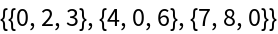### Scope (3)

Use on a sparse matrix:

 In:=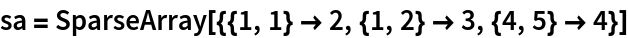Out=In:=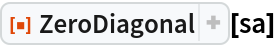Out=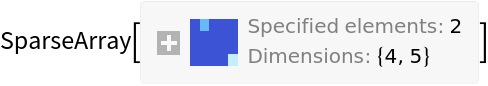Use on a non-square matrix with symbols:

 In:=Out=Use on a machine-precision matrix:

 In:=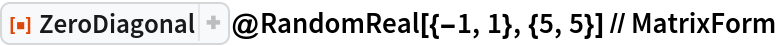Out=### Applications (1)

Create a random directed graph with no self-loops:

 In:=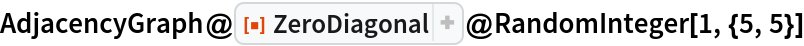Out=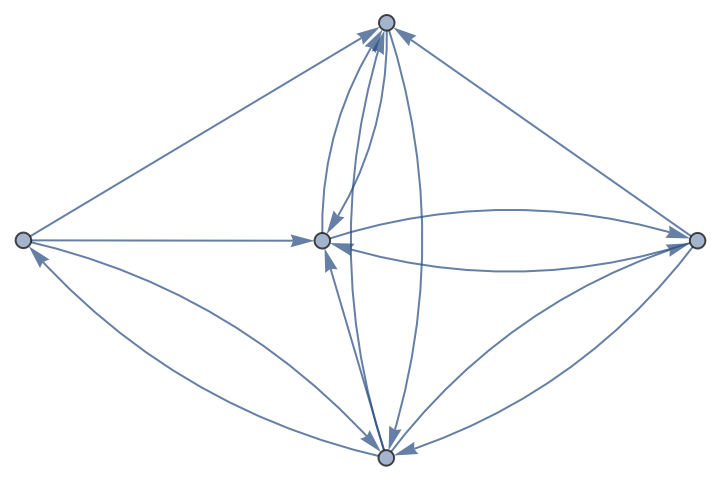Szabolcs Horvat

## Requirements

Wolfram Language 11.3 (March 2018) or above

## Version History

• 1.0.0 – 20 February 2019